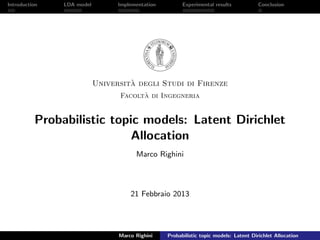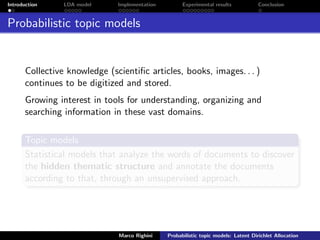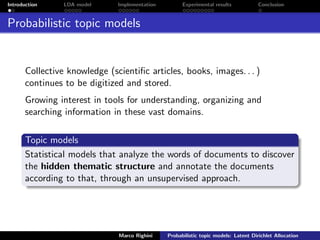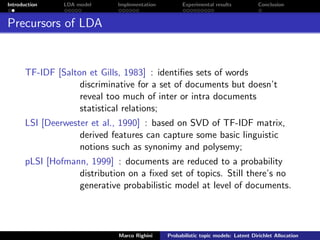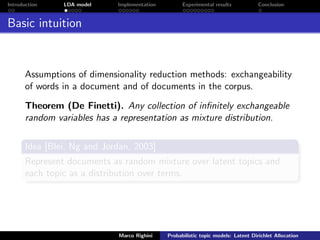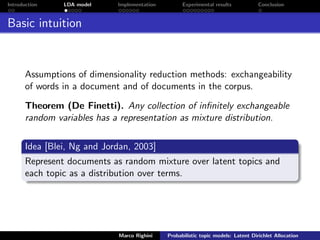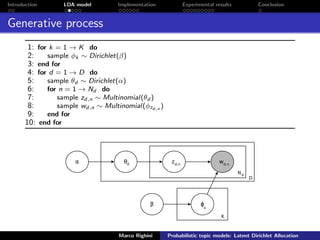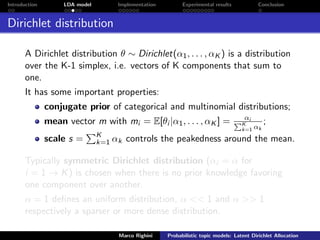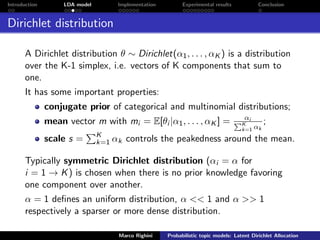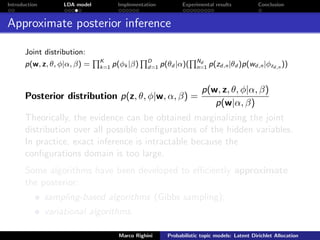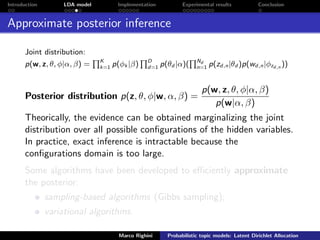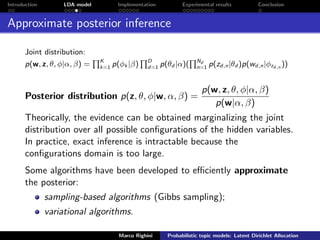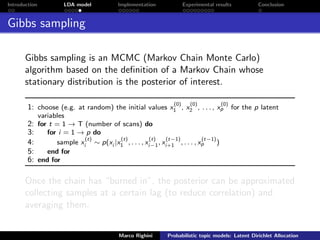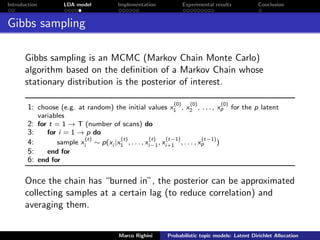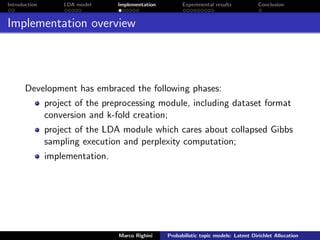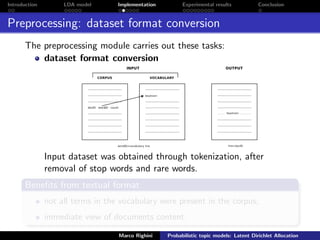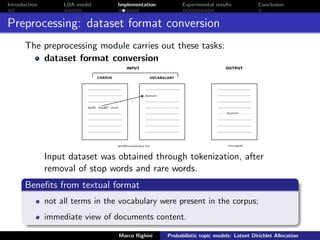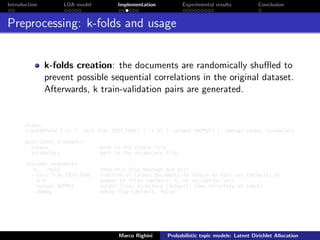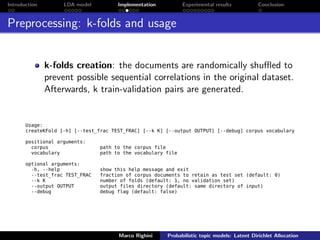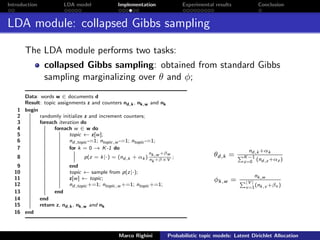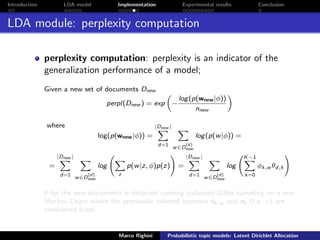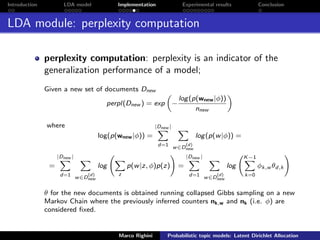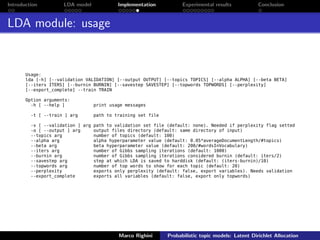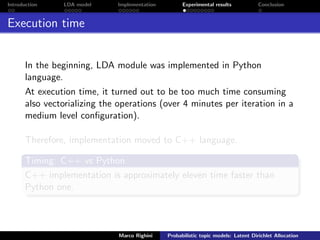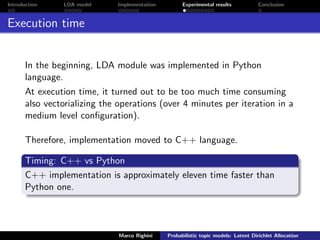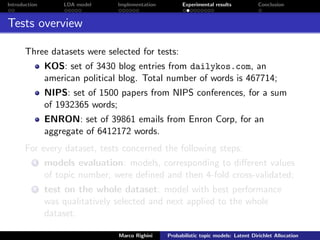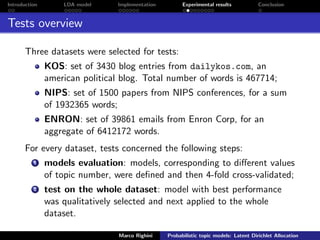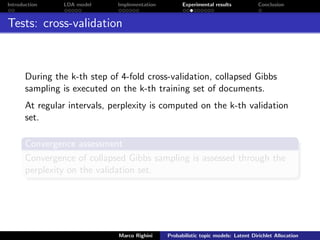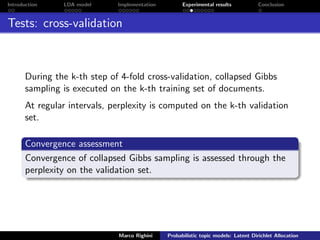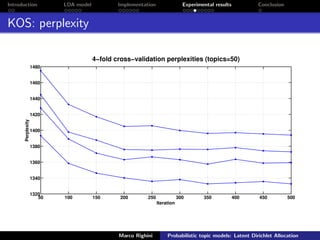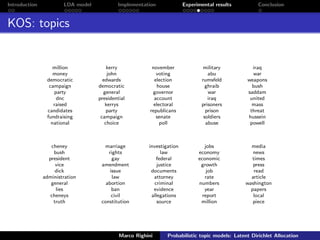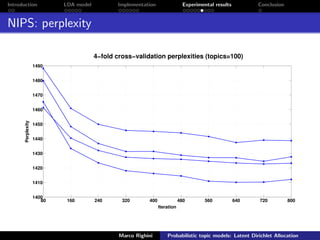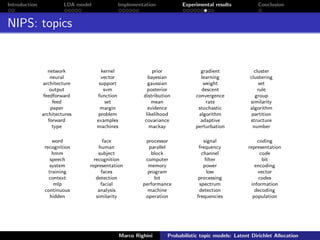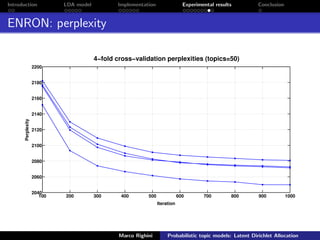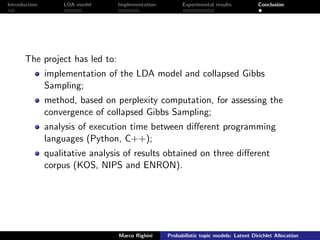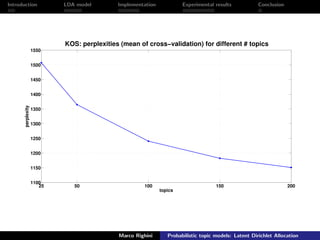1 of 37

### Latent Dirichlet Allocation

1. Introduction LDA model Implementation Experimental results Conclusion Universit`a degli Studi di Firenze Facolt`a di Ingegneria Probabilistic topic models: Latent Dirichlet Allocation Marco Righini 21 Febbraio 2013 Marco Righini Probabilistic topic models: Latent Dirichlet Allocation
2. Introduction LDA model Implementation Experimental results Conclusion Probabilistic topic models Collective knowledge (scientiﬁc articles, books, images. . . ) continues to be digitized and stored. Growing interest in tools for understanding, organizing and searching information in these vast domains. Topic models Statistical models that analyze the words of documents to discover the hidden thematic structure and annotate the documents according to that, through an unsupervised approach. Marco Righini Probabilistic topic models: Latent Dirichlet Allocation
3. Introduction LDA model Implementation Experimental results Conclusion Probabilistic topic models Collective knowledge (scientiﬁc articles, books, images. . . ) continues to be digitized and stored. Growing interest in tools for understanding, organizing and searching information in these vast domains. Topic models Statistical models that analyze the words of documents to discover the hidden thematic structure and annotate the documents according to that, through an unsupervised approach. Marco Righini Probabilistic topic models: Latent Dirichlet Allocation
4. Introduction LDA model Implementation Experimental results Conclusion Precursors of LDA TF-IDF [Salton et Gills, 1983] : identiﬁes sets of words discriminative for a set of documents but doesn’t reveal too much of inter or intra documents statistical relations; LSI [Deerwester et al., 1990] : based on SVD of TF-IDF matrix, derived features can capture some basic linguistic notions such as synonimy and polysemy; pLSI [Hofmann, 1999] : documents are reduced to a probability distribution on a ﬁxed set of topics. Still there’s no generative probabilistic model at level of documents. Marco Righini Probabilistic topic models: Latent Dirichlet Allocation
5. Introduction LDA model Implementation Experimental results Conclusion Basic intuition Assumptions of dimensionality reduction methods: exchangeability of words in a document and of documents in the corpus. Theorem (De Finetti). Any collection of inﬁnitely exchangeable random variables has a representation as mixture distribution. Idea [Blei, Ng and Jordan, 2003] Represent documents as random mixture over latent topics and each topic as a distribution over terms. Marco Righini Probabilistic topic models: Latent Dirichlet Allocation
6. Introduction LDA model Implementation Experimental results Conclusion Basic intuition Assumptions of dimensionality reduction methods: exchangeability of words in a document and of documents in the corpus. Theorem (De Finetti). Any collection of inﬁnitely exchangeable random variables has a representation as mixture distribution. Idea [Blei, Ng and Jordan, 2003] Represent documents as random mixture over latent topics and each topic as a distribution over terms. Marco Righini Probabilistic topic models: Latent Dirichlet Allocation
7. Introduction LDA model Implementation Experimental results Conclusion Generative process 1: for k = 1 → K do 2: sample φk ∼ Dirichlet(β) 3: end for 4: for d = 1 → D do 5: sample θd ∼ Dirichlet(α) 6: for n = 1 → Nd do 7: sample zd,n ∼ Multinomial(θd ) 8: sample wd,n ∼ Multinomial(φzd,n ) 9: end for 10: end for Marco Righini Probabilistic topic models: Latent Dirichlet Allocation
8. Introduction LDA model Implementation Experimental results Conclusion Dirichlet distribution A Dirichlet distribution θ ∼ Dirichlet(α1, . . . , αK ) is a distribution over the K-1 simplex, i.e. vectors of K components that sum to one. It has some important properties: conjugate prior of categorical and multinomial distributions; mean vector m with mi = E[θi |α1, . . . , αK ] = αi K k=1 αk ; scale s = K k=1 αk controls the peakedness around the mean. Typically symmetric Dirichlet distribution (αi = α for i = 1 → K) is chosen when there is no prior knowledge favoring one component over another. α = 1 deﬁnes an uniform distribution, α << 1 and α >> 1 respectively a sparser or more dense distribution. Marco Righini Probabilistic topic models: Latent Dirichlet Allocation
9. Introduction LDA model Implementation Experimental results Conclusion Dirichlet distribution A Dirichlet distribution θ ∼ Dirichlet(α1, . . . , αK ) is a distribution over the K-1 simplex, i.e. vectors of K components that sum to one. It has some important properties: conjugate prior of categorical and multinomial distributions; mean vector m with mi = E[θi |α1, . . . , αK ] = αi K k=1 αk ; scale s = K k=1 αk controls the peakedness around the mean. Typically symmetric Dirichlet distribution (αi = α for i = 1 → K) is chosen when there is no prior knowledge favoring one component over another. α = 1 deﬁnes an uniform distribution, α << 1 and α >> 1 respectively a sparser or more dense distribution. Marco Righini Probabilistic topic models: Latent Dirichlet Allocation
10. Introduction LDA model Implementation Experimental results Conclusion Approximate posterior inference Joint distribution: p(w, z, θ, φ|α, β) = K k=1 p(φk |β) D d=1 p(θd |α)( Nd n=1 p(zd,n|θd )p(wd,n|φzd,n )) Posterior distribution p(z, θ, φ|w, α, β) = p(w, z, θ, φ|α, β) p(w|α, β) Theorically, the evidence can be obtained marginalizing the joint distribution over all possible conﬁgurations of the hidden variables. In practice, exact inference is intractable because the conﬁgurations domain is too large. Some algorithms have been developed to eﬃciently approximate the posterior: sampling-based algorithms (Gibbs sampling); variational algorithms. Marco Righini Probabilistic topic models: Latent Dirichlet Allocation
11. Introduction LDA model Implementation Experimental results Conclusion Approximate posterior inference Joint distribution: p(w, z, θ, φ|α, β) = K k=1 p(φk |β) D d=1 p(θd |α)( Nd n=1 p(zd,n|θd )p(wd,n|φzd,n )) Posterior distribution p(z, θ, φ|w, α, β) = p(w, z, θ, φ|α, β) p(w|α, β) Theorically, the evidence can be obtained marginalizing the joint distribution over all possible conﬁgurations of the hidden variables. In practice, exact inference is intractable because the conﬁgurations domain is too large. Some algorithms have been developed to eﬃciently approximate the posterior: sampling-based algorithms (Gibbs sampling); variational algorithms. Marco Righini Probabilistic topic models: Latent Dirichlet Allocation
12. Introduction LDA model Implementation Experimental results Conclusion Approximate posterior inference Joint distribution: p(w, z, θ, φ|α, β) = K k=1 p(φk |β) D d=1 p(θd |α)( Nd n=1 p(zd,n|θd )p(wd,n|φzd,n )) Posterior distribution p(z, θ, φ|w, α, β) = p(w, z, θ, φ|α, β) p(w|α, β) Theorically, the evidence can be obtained marginalizing the joint distribution over all possible conﬁgurations of the hidden variables. In practice, exact inference is intractable because the conﬁgurations domain is too large. Some algorithms have been developed to eﬃciently approximate the posterior: sampling-based algorithms (Gibbs sampling); variational algorithms. Marco Righini Probabilistic topic models: Latent Dirichlet Allocation
13. Introduction LDA model Implementation Experimental results Conclusion Gibbs sampling Gibbs sampling is an MCMC (Markov Chain Monte Carlo) algorithm based on the deﬁnition of a Markov Chain whose stationary distribution is the posterior of interest. 1: choose (e.g. at random) the initial values x (0) 1 , x (0) 2 , . . . , x (0) p for the p latent variables 2: for t = 1 → T (number of scans) do 3: for i = 1 → p do 4: sample x (t) i ∼ p(xi |x (t) 1 , . . . , x (t) i−1, x (t−1) i+1 , . . . , x (t−1) p ) 5: end for 6: end for Once the chain has “burned in”, the posterior can be approximated collecting samples at a certain lag (to reduce correlation) and averaging them. Marco Righini Probabilistic topic models: Latent Dirichlet Allocation
14. Introduction LDA model Implementation Experimental results Conclusion Gibbs sampling Gibbs sampling is an MCMC (Markov Chain Monte Carlo) algorithm based on the deﬁnition of a Markov Chain whose stationary distribution is the posterior of interest. 1: choose (e.g. at random) the initial values x (0) 1 , x (0) 2 , . . . , x (0) p for the p latent variables 2: for t = 1 → T (number of scans) do 3: for i = 1 → p do 4: sample x (t) i ∼ p(xi |x (t) 1 , . . . , x (t) i−1, x (t−1) i+1 , . . . , x (t−1) p ) 5: end for 6: end for Once the chain has “burned in”, the posterior can be approximated collecting samples at a certain lag (to reduce correlation) and averaging them. Marco Righini Probabilistic topic models: Latent Dirichlet Allocation
15. Introduction LDA model Implementation Experimental results Conclusion Implementation overview Development has embraced the following phases: project of the preprocessing module, including dataset format conversion and k-fold creation; project of the LDA module which cares about collapsed Gibbs sampling execution and perplexity computation; implementation. Marco Righini Probabilistic topic models: Latent Dirichlet Allocation
16. Introduction LDA model Implementation Experimental results Conclusion Preprocessing: dataset format conversion The preprocessing module carries out these tasks: dataset format conversion Input dataset was obtained through tokenization, after removal of stop words and rare words. Beneﬁts from textual format not all terms in the vocabulary were present in the corpus; immediate view of documents content. Marco Righini Probabilistic topic models: Latent Dirichlet Allocation
17. Introduction LDA model Implementation Experimental results Conclusion Preprocessing: dataset format conversion The preprocessing module carries out these tasks: dataset format conversion Input dataset was obtained through tokenization, after removal of stop words and rare words. Beneﬁts from textual format not all terms in the vocabulary were present in the corpus; immediate view of documents content. Marco Righini Probabilistic topic models: Latent Dirichlet Allocation
18. Introduction LDA model Implementation Experimental results Conclusion Preprocessing: k-folds and usage k-folds creation: the documents are randomically shuﬄed to prevent possible sequential correlations in the original dataset. Afterwards, k train-validation pairs are generated. Usage: createKFold [-h] [--test_frac TEST_FRAC] [--k K] [--output OUTPUT] [--debug] corpus vocabulary positional arguments: corpus path to the corpus file vocabulary path to the vocabulary file optional arguments: -h, --help show this help message and exit --test_frac TEST_FRAC fraction of corpus documents to retain as test set (default: 0) --k K number of folds (default: 1, no validation set) --output OUTPUT output files directory (default: same directory of input) --debug debug flag (default: false) Marco Righini Probabilistic topic models: Latent Dirichlet Allocation
19. Introduction LDA model Implementation Experimental results Conclusion Preprocessing: k-folds and usage k-folds creation: the documents are randomically shuﬄed to prevent possible sequential correlations in the original dataset. Afterwards, k train-validation pairs are generated. Usage: createKFold [-h] [--test_frac TEST_FRAC] [--k K] [--output OUTPUT] [--debug] corpus vocabulary positional arguments: corpus path to the corpus file vocabulary path to the vocabulary file optional arguments: -h, --help show this help message and exit --test_frac TEST_FRAC fraction of corpus documents to retain as test set (default: 0) --k K number of folds (default: 1, no validation set) --output OUTPUT output files directory (default: same directory of input) --debug debug flag (default: false) Marco Righini Probabilistic topic models: Latent Dirichlet Allocation
20. Introduction LDA model Implementation Experimental results Conclusion LDA module: collapsed Gibbs sampling The LDA module performs two tasks: collapsed Gibbs sampling: obtained from standard Gibbs sampling marginalizing over θ and φ; Data: words w ∈ documents d Result: topic assignments z and counters nd,k, nk,w and nk 1 begin 2 randomly initialize z and increment counters; 3 foreach iteration do 4 foreach w ∈ w do 5 topic ← z[w]; 6 nd,topic -=1; ntopic,w -=1; ntopic -=1; 7 for k = 0 → K-1 do 8 p(z = k|·) = (nd,k + αk ) nk,w +βw nk +β×V ; 9 end 10 topic ← sample from p(z|·); 11 z[w] ← topic; 12 nd,topic +=1; ntopic,w +=1; ntopic +=1; 13 end 14 end 15 return z, nd,k, nk,w and nk 16 end θd,k = nd,k +αk K−1 z=0 (nd,z +αz ) φk,w = nk,w |V | v=1(nk,v +βv ) Marco Righini Probabilistic topic models: Latent Dirichlet Allocation
21. Introduction LDA model Implementation Experimental results Conclusion LDA module: perplexity computation perplexity computation: perplexity is an indicator of the generalization performance of a model; Given a new set of documents Dnew perpl(Dnew ) = exp − log(p(wnew|φ)) nnew where log(p(wnew|φ)) = |Dnew | d=1 w∈D (d) new log(p(w|φ)) = = |Dnew | d=1 w∈D (d) new log z p(w|z, φ)p(z) = |Dnew | d=1 w∈D (d) new log K−1 k=0 φk,w θd,k θ for the new documents is obtained running collapsed Gibbs sampling on a new Markov Chain where the previously inferred counters nk,w and nk (i.e. φ) are considered ﬁxed. Marco Righini Probabilistic topic models: Latent Dirichlet Allocation
22. Introduction LDA model Implementation Experimental results Conclusion LDA module: perplexity computation perplexity computation: perplexity is an indicator of the generalization performance of a model; Given a new set of documents Dnew perpl(Dnew ) = exp − log(p(wnew|φ)) nnew where log(p(wnew|φ)) = |Dnew | d=1 w∈D (d) new log(p(w|φ)) = = |Dnew | d=1 w∈D (d) new log z p(w|z, φ)p(z) = |Dnew | d=1 w∈D (d) new log K−1 k=0 φk,w θd,k θ for the new documents is obtained running collapsed Gibbs sampling on a new Markov Chain where the previously inferred counters nk,w and nk (i.e. φ) are considered ﬁxed. Marco Righini Probabilistic topic models: Latent Dirichlet Allocation
23. Introduction LDA model Implementation Experimental results Conclusion LDA module: usage Usage: lda [-h] [--validation VALIDATION] [--output OUTPUT] [--topics TOPICS] [--alpha ALPHA] [--beta BETA] [--iters ITERS] [--burnin BURNIN] [--savestep SAVESTEP] [--topwords TOPWORDS] [--perplexity] [--export_complete] --train TRAIN Option arguments: -h [ --help ] print usage messages -t [ --train ] arg path to training set file -v [ --validation ] arg path to validation set file (default: none). Needed if perplexity flag setted -o [ --output ] arg output files directory (default: same directory of input) --topics arg number of topics (default: 100) --alpha arg alpha hyperparameter value (default: 0.05*averageDocumentLength/#topics) --beta arg beta hyperparameter value (default: 200/#wordsInVocabulary) --iters arg number of Gibbs sampling iterations (default: 1000) --burnin arg number of Gibbs sampling iterations considered burnin (default: iters/2) --savestep arg step at which LDA is saved to harddisk (default: (iters-burnin)/10) --topwords arg number of top words to show for each topic (default: 20) --perplexity exports only perplexity (default: false, export variables). Needs validation --export_complete exports all variables (default: false, export only topwords) Marco Righini Probabilistic topic models: Latent Dirichlet Allocation
24. Introduction LDA model Implementation Experimental results Conclusion Execution time In the beginning, LDA module was implemented in Python language. At execution time, it turned out to be too much time consuming also vectorializing the operations (over 4 minutes per iteration in a medium level conﬁguration). Therefore, implementation moved to C++ language. Timing: C++ vs Python C++ implementation is approximately eleven time faster than Python one. Marco Righini Probabilistic topic models: Latent Dirichlet Allocation
25. Introduction LDA model Implementation Experimental results Conclusion Execution time In the beginning, LDA module was implemented in Python language. At execution time, it turned out to be too much time consuming also vectorializing the operations (over 4 minutes per iteration in a medium level conﬁguration). Therefore, implementation moved to C++ language. Timing: C++ vs Python C++ implementation is approximately eleven time faster than Python one. Marco Righini Probabilistic topic models: Latent Dirichlet Allocation
26. Introduction LDA model Implementation Experimental results Conclusion Tests overview Three datasets were selected for tests: KOS: set of 3430 blog entries from dailykos.com, an american political blog. Total number of words is 467714; NIPS: set of 1500 papers from NIPS conferences, for a sum of 1932365 words; ENRON: set of 39861 emails from Enron Corp, for an aggregate of 6412172 words. For every dataset, tests concerned the following steps: 1 models evaluation: models, corresponding to diﬀerent values of topic number, were deﬁned and then 4-fold cross-validated; 2 test on the whole dataset: model with best performance was qualitatively selected and next applied to the whole dataset. Marco Righini Probabilistic topic models: Latent Dirichlet Allocation
27. Introduction LDA model Implementation Experimental results Conclusion Tests overview Three datasets were selected for tests: KOS: set of 3430 blog entries from dailykos.com, an american political blog. Total number of words is 467714; NIPS: set of 1500 papers from NIPS conferences, for a sum of 1932365 words; ENRON: set of 39861 emails from Enron Corp, for an aggregate of 6412172 words. For every dataset, tests concerned the following steps: 1 models evaluation: models, corresponding to diﬀerent values of topic number, were deﬁned and then 4-fold cross-validated; 2 test on the whole dataset: model with best performance was qualitatively selected and next applied to the whole dataset. Marco Righini Probabilistic topic models: Latent Dirichlet Allocation
28. Introduction LDA model Implementation Experimental results Conclusion Tests: cross-validation During the k-th step of 4-fold cross-validation, collapsed Gibbs sampling is executed on the k-th training set of documents. At regular intervals, perplexity is computed on the k-th validation set. Convergence assessment Convergence of collapsed Gibbs sampling is assessed through the perplexity on the validation set. Marco Righini Probabilistic topic models: Latent Dirichlet Allocation
29. Introduction LDA model Implementation Experimental results Conclusion Tests: cross-validation During the k-th step of 4-fold cross-validation, collapsed Gibbs sampling is executed on the k-th training set of documents. At regular intervals, perplexity is computed on the k-th validation set. Convergence assessment Convergence of collapsed Gibbs sampling is assessed through the perplexity on the validation set. Marco Righini Probabilistic topic models: Latent Dirichlet Allocation
30. Introduction LDA model Implementation Experimental results Conclusion KOS: perplexity 50 100 150 200 250 300 350 400 450 500 1320 1340 1360 1380 1400 1420 1440 1460 1480 Iteration Perplexity 4−fold cross−validation perplexities (topics=50) Marco Righini Probabilistic topic models: Latent Dirichlet Allocation
31. Introduction LDA model Implementation Experimental results Conclusion KOS: topics million money democratic campaign party dnc raised candidates fundraising national kerry john edwards democratic general presidential kerrys party campaign choice november voting election house governor account electoral republicans senate poll military abu rumsfeld ghraib war iraq prisoners prison soldiers abuse iraq war weapons bush saddam united mass threat hussein powell cheney bush president vice dick administration general lies cheneys truth marriage rights gay amendment issue law abortion ban civil constitution investigation law federal justice documents attorney criminal evidence allegations source jobs economy economic growth job rate numbers year report million media news times press read article washington papers local piece Marco Righini Probabilistic topic models: Latent Dirichlet Allocation
32. Introduction LDA model Implementation Experimental results Conclusion NIPS: perplexity 80 160 240 320 400 480 560 640 720 800 1400 1410 1420 1430 1440 1450 1460 1470 1480 1490 Iteration Perplexity 4−fold cross−validation perplexities (topics=100) Marco Righini Probabilistic topic models: Latent Dirichlet Allocation
33. Introduction LDA model Implementation Experimental results Conclusion NIPS: topics network neural architecture output feedforward feed paper architectures forward type kernel vector support svm function set margin problem examples machines prior bayesian gaussian posterior distribution mean evidence likelihood covariance mackay gradient learning weight descent convergence rate stochastic algorithm adaptive perturbation cluster clustering set rule group similarity algorithm partition structure number word recognition hmm speech system training context mlp continuous hidden face human subject recognition representation faces detection facial analysis similarity processor parallel block computer memory program bit performance machine operation signal frequency channel ﬁlter power low processing spectrum detection frequencies coding representation code bit encoding vector codes information decoding population Marco Righini Probabilistic topic models: Latent Dirichlet Allocation
34. Introduction LDA model Implementation Experimental results Conclusion ENRON: perplexity 100 200 300 400 500 600 700 800 900 1000 2040 2060 2080 2100 2120 2140 2160 2180 2200 Iteration Perplexity 4−fold cross−validation perplexities (topics=50) Marco Righini Probabilistic topic models: Latent Dirichlet Allocation
35. Introduction LDA model Implementation Experimental results Conclusion ENRON: topics energy program plant air emission environmental demand wind generation renewable project permit station facility construction site water unit area facilities company stock ﬁnancial billion dynegy investor shares earning analyst trading customer cost rate contract credit rates pay amount sce period page court labor employees law worker union federal employer rules access user password center account web link help message site student business school program university haas class mba event berkeley american world attack country government war article bush city international travel hotel roundtrip fares special miles city ticket oﬀer deal free click online oﬀer receive link special web visit account Marco Righini Probabilistic topic models: Latent Dirichlet Allocation
36. Introduction LDA model Implementation Experimental results Conclusion The project has led to: implementation of the LDA model and collapsed Gibbs Sampling; method, based on perplexity computation, for assessing the convergence of collapsed Gibbs Sampling; analysis of execution time between diﬀerent programming languages (Python, C++); qualitative analysis of results obtained on three diﬀerent corpus (KOS, NIPS and ENRON). Marco Righini Probabilistic topic models: Latent Dirichlet Allocation
37. Introduction LDA model Implementation Experimental results Conclusion 25 50 100 150 200 1100 1150 1200 1250 1300 1350 1400 1450 1500 1550 topics perplexity KOS: perplexities (mean of cross−validation) for different # topics Marco Righini Probabilistic topic models: Latent Dirichlet Allocation